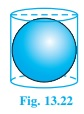Question

# A right circular cylinder just encloses a sphere of radius $r$ (see fig.). Find (i) surface area of the sphere, (ii) curved surface area of the cylinder, (iii) ratio of the areas obtained in(i), and (ii)Fig. 13.22

Open in App
Solution

## (i) Surface area of a sphere $=4{\mathrm{\pi r}}^{2}$, where r is the radius of the sphere.(ii) As the Height of the cylinder, $h=r+r=2r$And Radius of the cylinder $=r$CSA of cylinder formula $=2\mathrm{\pi rh}=2\mathrm{\pi r}\left(2\mathrm{r}\right)$ (using the value of $h$) $=4{\mathrm{\pi r}}^{2}$(iii) Ratio between areas $=$$\frac{surfaceareaofthesphere}{CSAofcylinder}$ $=\frac{4{r}^{2}}{4{r}^{2}}=\frac{1}{1}$Therefore, the ratio of the areas obtained in (i) and (ii) is $1:1$.Suggest Corrections0Similar questions